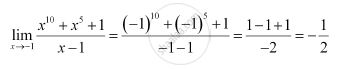CBSE (Science) Class 11CBSE
Share
Notifications

View all notifications
Books Shortlist
Your shortlist is empty

# Solution for Evaluate the Given Limit: Lim_(X-> -1) (X^10 + X^5 +1)/(X -1) - CBSE (Science) Class 11 - Mathematics

Login
Create free account

Forgot password?
ConceptIntuitive Idea of Derivatives

#### Question

Evaluate the Given limit: lim_(x-> -1) (x^10 + x^5 +1)/(x -1)

#### SolutionIs there an error in this question or solution?

#### APPEARS IN

NCERT Solution for Mathematics Textbook for Class 11 (2013 to Current)
Chapter 13: Limits and Derivatives
Q: 5 | Page no. 301
Solution Evaluate the Given Limit: Lim_(X-> -1) (X^10 + X^5 +1)/(X -1) Concept: Intuitive Idea of Derivatives.
S